# How to Auto Change the Shape and Size Based on a Specified Cell Value in Excel?

When we want to change the size of a specific shape in Excel based on the value present in any cell, we can use VB code to do it. Read this tutorial to learn how you can automatically change the shape and size based on a specified cell value in Excel. Here we will have two parts: the first is to change only one shape, and the other is to change multiple shapes at once.

## Auto Change the Shape and Size Based on a Specified Cell Value for a Single Shape

Here we will add the VBA code to the sheet to complete our task. Let us see a simple process to understand how we can automatically change shape size based on a specified cell value for a single shape.

Step 1

Let us consider an Excel sheet similar to the one shown in the below image.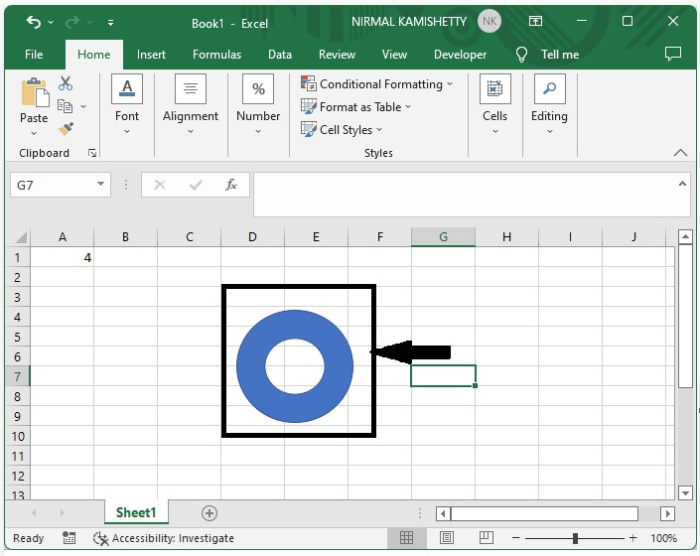Now right-click on the sheet name and select view code to open the VB application, and type the programme into the text box as shown in the below image.

### Program

Private Sub Worksheet_Change(ByVal Target As Range)
'Updated BY Nirmal
On Error Resume Next
If Target.Row = 1 And Target.Column = 1 Then
Call SizeCircle("Hollow 1", Val(Target.Value))
End If
End Sub
Sub SizeCircle(Name As String, Diameter)
Dim xCenterX As Single
Dim xCenterY As Single
Dim xCircle As Shape
Dim xDiameter As Single
On Error GoTo ExitSub
xDiameter = Diameter
If xDiameter > 10 Then xDiameter = 10
If xDiameter < 1 Then xDiameter = 1
Set xCircle = ActiveSheet.Shapes(Name)
With xCircle
xCenterX = .Left + (.Width / 2)
xCenterY = .Top + (.Height / 2)
.Width = Application.CentimetersToPoints(xDiameter)
.Height = Application.CentimetersToPoints(xDiameter)
.Left = xCenterX - (.Width / 2)
.Top = xCenterY - (.Height / 2)
End With
ExitSub:
End Sub


The name of the shape in Excel is "Hollow 1" in the code, and "row = 1" and "column = 1" represent cell A1.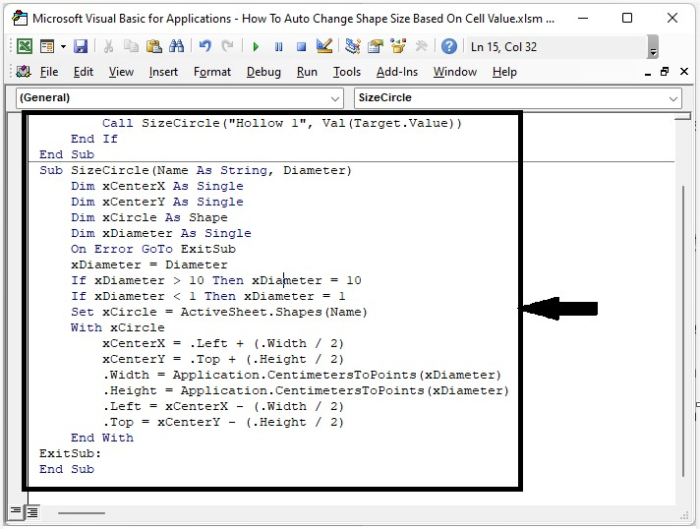Step 2

Save the sheet as a VBA template before exiting the VBA application with Alt + Q. Then every time we change the value in a cell, the shape of the cell changes automatically, as shown in the below image.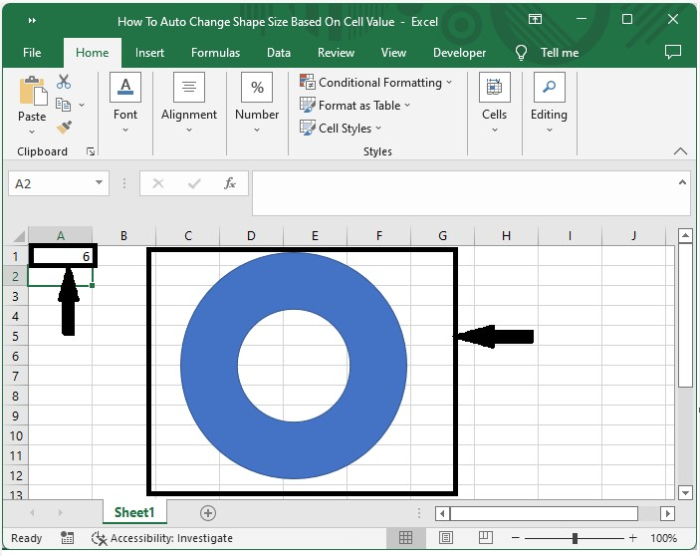## Auto Change the Shape and Size Based on a Specified Cell Value for Multiple Shapes

Here we will add VBA code to the sheet to complete our task. Let's see a simple process to understand how we can automatically change shape size based on a specified cell value for multiple shapes.

Step 1

Let us consider an Excel sheet similar to the one shown in the below image.

Now right-click on the sheet name and select View Code to open the VBA application, and type the programme into the text box as shown in the below image.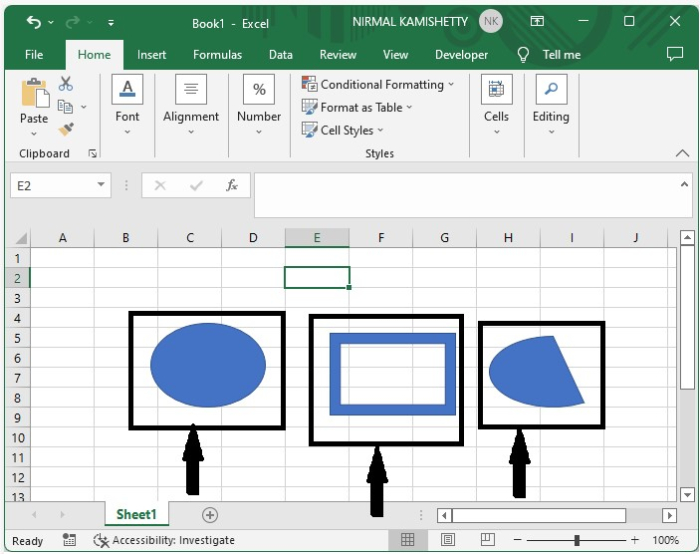### Program

Private Sub Worksheet_Change(ByVal Target As Range)
On Error Resume Next
If Target.CountLarge = 1 Then
Call SizeCircle("Oval 1", Val(Target.Value))
Call SizeCircle("Frame 2", Val(Target.Value))
Call SizeCircle("Chord 3", Val(Target.Value))
End If
End If
End Sub

Sub SizeCircle(Name As String, Diameter)
Dim xCenterX As Single
Dim xCenterY As Single
Dim xCircle As Shape
Dim xDiameter As Single
On Error GoTo ExitSub
xDiameter = Diameter
If xDiameter > 20 Then xDiameter = 20
If xDiameter < 1 Then xDiameter = 1
Set xCircle = ActiveSheet.Shapes(Name)
With xCircle
xCenterX = .Left + (.Width / 2)
xCenterY = .Top + (.Height / 2)
.Width = Application.CentimetersToPoints(xDiameter)
.Height = Application.CentimetersToPoints(xDiameter)
.Left = xCenterX - (.Width / 2)
.Top = xCenterY - (.Height / 2)
End With
ExitSub:
End Sub


In the code, Oval 1, Frame 2, and Chord 3 are the names of shapes, and cells A1, B1, and C2 decide their sizes, respectively.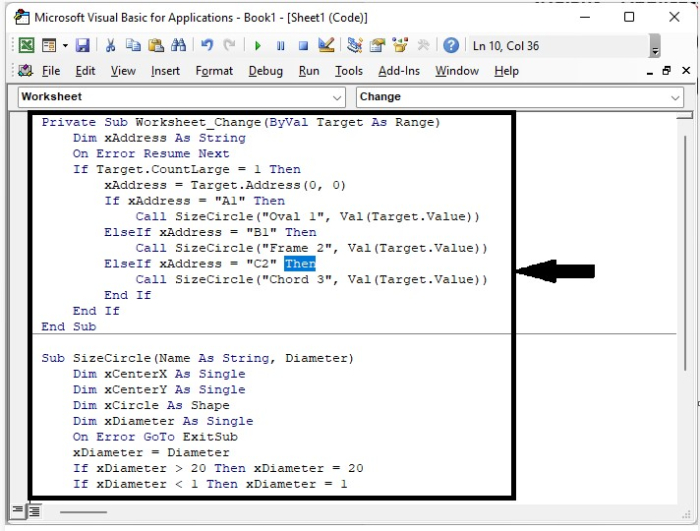Step 2

Save the sheet as a VBA template before exiting the VBA application with Alt + Q. Then every time we change the value in a cell, the shape of the cell changes automatically, as shown in the below image.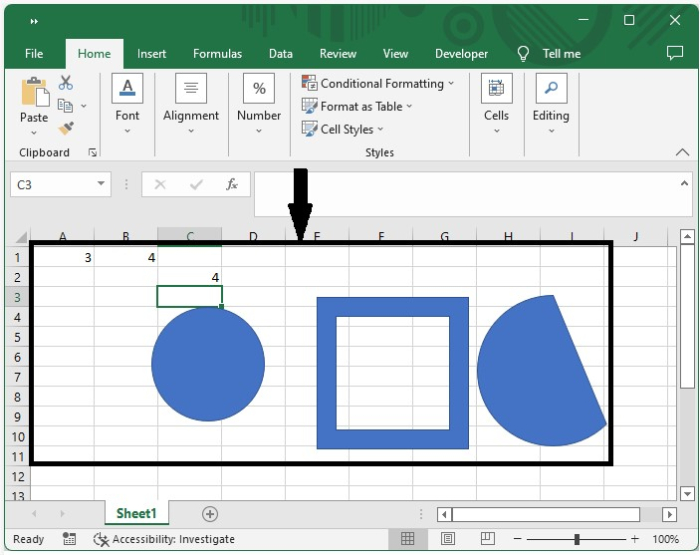## Conclusion

In this tutorial, we used a simple example to demonstrate how we can automatically change the shape and size based on a specified cell value for single and multiple shapes.

Updated on: 03-Jan-2023

1K+ Views